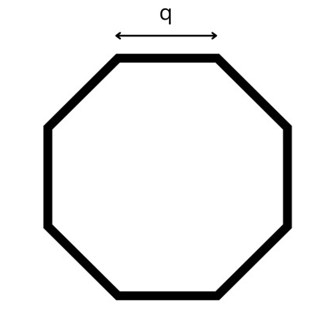# Swift Program to Calculate Area of Octagon

This tutorial will discuss how to write swift program to calculate area of octagon.

An octagon is a two-dimensional shape with 8 sides and also consists of eight interior and exterior angles. The total amount of space enclosed inside the octagon is known as the area of the octagon.## Formula:

Following is the formula of the area of the octagon −

Area = 2 *(side)2*(1+√2)


Below is a demonstration of the same −

Input

Suppose our given input is −

side = 15


Output

The desired output would be −

Area of octagon = 1086.3961030678927


## Algorithm

Following is the algorithm −

Step 1- Create a function with return value.

Step 2- Find the area of the octagon using the following formula:

return 2 * q * q * (1+sqrt(2))


Step 3- Calling the function and pass the side in the circle as a parameter.

Step 4- Print the output.

## Example

The following program shows how to calculate the area of octagon.

import Foundation
import Glibc

// Creating a function to find the area of octagon
func octagonArea(q:Double) -> Double{
return 2 * q * q * (1+sqrt(2))
}
var num = 10.0
print("Length of the side is", num)
print("Area of the octagon:", octagonArea(q:num))


## Output

Length of the side is 10.0
Area of the octagon: 482.84271247461896


Here, in the above program we create a function which return the area of the octagon using the following formula −

return 2 * q * q * (1+sqrt(2))


Here, we use sqrt() function to find the square root of 2.

Updated on: 30-Nov-2022

77 Views